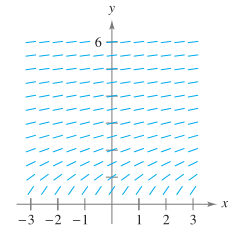Chapter 6.1, Problem 66E

Chapter
Section
Textbook Problem

Slope Field Use the slope field for the differential equation y ′ = 1 / y , where y > 0 , to sketchthe graph of the solution that satisfies each given initial condition. Then make a conjecture aboutthe behavior of a particular solution of y ′ = 1 / y as x → ∞ . To print an enlarged copy of the graph,go to MathGraphs.com.a). ( 0 , 1 ) b). ( 1 , 1 )

a)

To determine

To Graph: The solution that satisfies the given initial condition and then make a conjecture about the behavior of a particular solution of y=1/y as x.

Explanation

Given: The differential equation y=1/y, where y>0 and the point (0,1). The slope field for the differential equationis represented by the graph.

Graph:

We start by creating a table showing the slopes at several points.

The table shown below is a smallsample.

The slopes at many other points should be calculated to get a representative slope field.

 x -2 -2 -1 -1 0 0 1 1 2 2 y -1 1 -1 1 -1 1 -1 1 -1 1 y′=1/y -1 1 -1 1 -1 1 -1 1 -1 1

Now, we draw line segments at the points with their respective slopes as shown below.

On solving the differential equation y=1/y, we have

dydx=1y

ydy=dx

So, Integrating both sides, we get

ydy=(dx)

12y2=x<

b)

To determine

To Graph: The solution that satisfies the given initial condition and then make a conjecture about the behavior of a particular solution of y=1/y as x.

Still sussing out bartleby?

Check out a sample textbook solution.

See a sample solution

The Solution to Your Study Problems

Bartleby provides explanations to thousands of textbook problems written by our experts, many with advanced degrees!

Get Started

Find more solutions based on key concepts